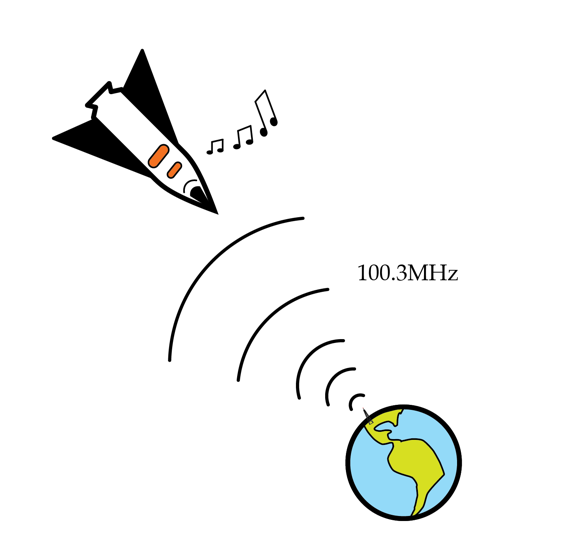# Space oddity radio

I'm in a spaceship very far away from Earth but traveling straight towards earth with a speed v. It's boring out here, so I decide to try and tune in to some of my favorite earthly radio stations. I remember that my favorite station has a frequency of 100.3 MHz and so tune my radio to exactly this frequency. Amazingly, I hear the radio station just like I do on Earth! How fast is my spaceship going in m/s? (Hint: it's not that fast... I think I should check whether my engines are on).Details and assumptions

• Photons of electromagnetic radiation have an intrinsic kinetic energy related to their frequency by $E=hf$ where $h$ is Planck's constant.
• The gravitational interaction between photons and Earth can be treated via usual Newtonian gravity and $E=mc^2$ to convert between energy and mass.
• The total energy of the photons is conserved.
• The Earth can be treated as a sphere of radius 6370 km and mass $6 \times 10^{24}~kg$. You can ignore rotation of the earth.
• The speed of light is $3 \times 10^8~m/s$.
• Assume the earth is at rest.
×

Problem Loading...

Note Loading...

Set Loading...